# Brimrose AOTF-NIR光谱法测定低密度聚乙烯的密度和熔融指数

I. 目的

II. 简介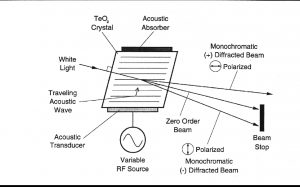III.  Methodology

IV.Results

1. Spectra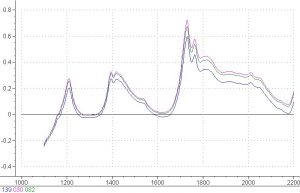Figure 2.  Absorbance spectra of some polyethylene samples.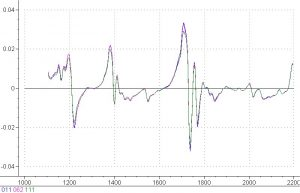Figure 3.  First derivative spectra of some polyethylene samples.

#### 2.      Regressions and Modeling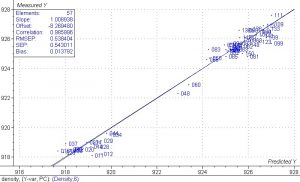#### Figure 4.  PLS 1 regression model for density in polyethylene samples.

This PLS 1 regression plot for density in the polyethylene samples shows excellent correlation between the measured and predicted values.  The two distinct data sets in this plot are due to two different grades of material.  Samples 048 and 060 are two transition samples.  The data points create a good line of best fit and the correlation coefficient value of 0.985 is very high.   The SEP is equal to 0.54, which proves that the model from this regression will be able to accurately predict density in polyethylene from spectral data.  The SEP is well within the standard deviation target value of 6%.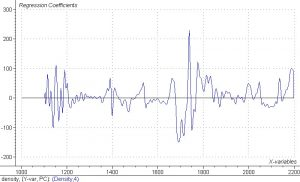Figure 5.   Regression coefficients for PLS 1 analysis of density in polyethylene samples.
The regression coefficients plot for the PLS 1 regression for density shows that most of the information for the regression comes from the wavelength regions from 1680nm to 1740nm.  This area corresponds to the first overtone of the C-H stretch and is the region where one would expect to see spectral changes corresponding to changes in density.  Density in polymers corresponds to changes in crystallization and this is what causes the changes in the spectral data.  The fact that the first derivative data shows the greatest amount of change in the wavelength regions from 1680nm to 1740nm confirms that changes in density can be quantified using spectral data from these wavelength regions and a calibration model.
Because there was a wide range of values for melt index, it was decided to split the calibration for melt index into a low melt index calibration and a high melt index calibration.
The range of values for low melt index was from 0.7 to 2.0 and the range for low melt index was from 2.0 to 95.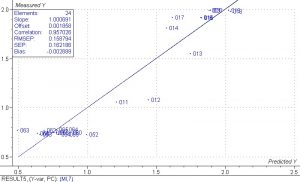Figure 6.  PLS 1 regression model for low melt index in polyethylene samples.   SEP is equal to 0.16 and the correlation coefficient is equal to 0.957.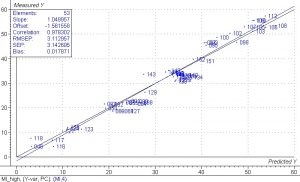Figure 7.  PLS 1 regression model for high melt index in polyethylene samples.  SEP is equal to 3.14 and the correlation coefficient is equal to 0.978.
The results for these regressions show that it is quite feasible to measure melt index in polyethylene using spectral data.   There was a 6% target for relative error and both the high and low melt index regressions were well within this value.  One high melt index sample had an extremely high value and was left out of the calculation as an outlier.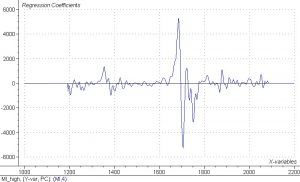Figure 8.   Regression coefficients for PLS 1 analysis of high melt flow index in polyethylene samples.

As was the case with density, the regression coefficients indicate that most of the information for the high melt flow index regression comes from the 1680nm to 1740nm wavelength range, which is the first overtone of the C-H stretch.  The small amount of noise in the regression coefficients indicates that there is no overfitting of the data.

Parameter SEP Correlation Coefficient
Density 0.54 0.986
0.006 0.982
###### High Melt Index
3.14 0.978

Table 1.  Summary of SEPs and correlation coefficients from PLS 1 regressions for density, low melt index, and high melt index in polyethylene samples.
V. Conclusions and Recommendations
This feasibility study has shown that the Brimrose Free Space spectrometer can be used to measure density and melt index in low density polyethylene.  The low value of SEP and the high value of correlation coefficients are well within the standard deviation of 6%.  Previous experience has shown that the use of local models in the case of melt index lowers the SEP even more.  These samples were measured in the laboratory using a rotating cup assembly and in-line measurement should show even better results.  Spectral rejection software developed by Brimrose will prove invaluable in this application.  This software will only average spectra when there is sufficient material in front of the window and will reject spectra when the amount of material is insufficient.   A self-cleaning window will ensure that enough good spectra can be taken.  It is recommended that Brimrose install a spectrometer to conduct in-line tests of low density polyethylene which can be used to create a model that will allow for continuous in-line testing of polyethylene for density and melt flow index.